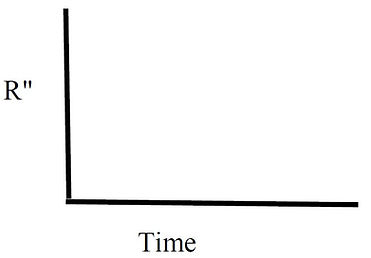###### Workshop #5 - Transient Thermal Resistance Introduction

One very useful model of transient heat transfer is the semi-infinite solid. This combines the effects of the thermal resistance of the material along with the thermal capacitance of the material to absorb thermal energy. The main assumptions are that the material is thick (semi-infinite) and that heat transfer is one-dimensional. Look in a heat transfer text to find the theory of semi-infinite heat transfer. Although the mathematics involves partial differential equation solutions, the results are rather simple. If a constant temperature source Ts is applied to the surface of the material without any contact resistance, the resulting heat flux into the surface is

The initial temperature of the material is a uniform Ti and the surface temperature change happens at time t = 0. The properties of the material are thermal conductivity k, specific heat C, and density ρ.

The required thickness is a function of time. Conduction heat transfer is a diffusion process that propagates into the material as time increases. Consequently, the thickness to appear to be infinitely thick increases with time. A good approximation for this thickness L is

where the thermal diffusivity α = k/ρC. It is an important property for transient heat transfer problems that indicates how fast heat transfers into a material.

Here are properties of concrete and carpet that will be used in this workshop. Calculate the remaining values of thermal diffusivity and penetration depth for the semi-infinite solution:

Another way to view this problem is in terms of the thermal resistance of the material R". It is defined as the ratio of the temperature difference to the heat flux.

Determine the theoretical value of thermal resistance for the constant surface temperature semi-infinite solution,

R"

How does it vary with time?

Sketch how it should vary.###### Workshop #5 - Transient Thermal Resistance Results

The goal of this workshop is to look at the heat transfer response of two materials with very different properties – concrete floor and carpet. Use your hand as a heat source and the heat flux sensor to measure the surface heat flux and surface temperature as a function of time. The goal is to see how the properties of these different materials affect your results.

a) Put the sensor on a concrete floor, start the data acquisition and then firmly place your hand entirely over the sensor for           about 20 seconds.

b) Now put the sensor directly onto a carpet and repeat.

From your saved data files, plot the temperature as a function of time for both materials on the same graph and compare. Do the same for the heat flux on a second graph. Model the solid material as a semi-infinite solid assuming that the sensor temperature is the surface temperature Ts. For the initial temperature of the material Ti use the sensor temperature before you apply your hand to create the thermal event. Use the measured heat flux with these measured temperatures to calculate the apparent thermal resistance in each material as a function of time, Rʺ = (Ts – Ti)/qʺ. Plot for the concrete and the carpet on the same graph for the time period where your hand is on the sensor. Attach the three plots (temperature, heat flux and thermal resistance) to hand in.

1. Based on your calculations for the thermal penetration depth L are the materials you tested sufficiently thick to assume           they are “semi-infinite”? Why?

2. Is the graph of your results for thermal resistance R" similar in shape to the theory shown in the introduction to Workshop           5?

3. Physically (apart from the equations) why does the thermal resistance change with time?

4. How does the value of thermal resistance vary between the two materials? Why? (look at thermal conductivity values)

5. Which material has the higher heat flux? Why?

6. Which material has the larger change in temperature? Why?

7. Even though the initial temperature of the carpet and the concrete are nearly the same, why does concrete feel colder                than the carpet (insulation)?